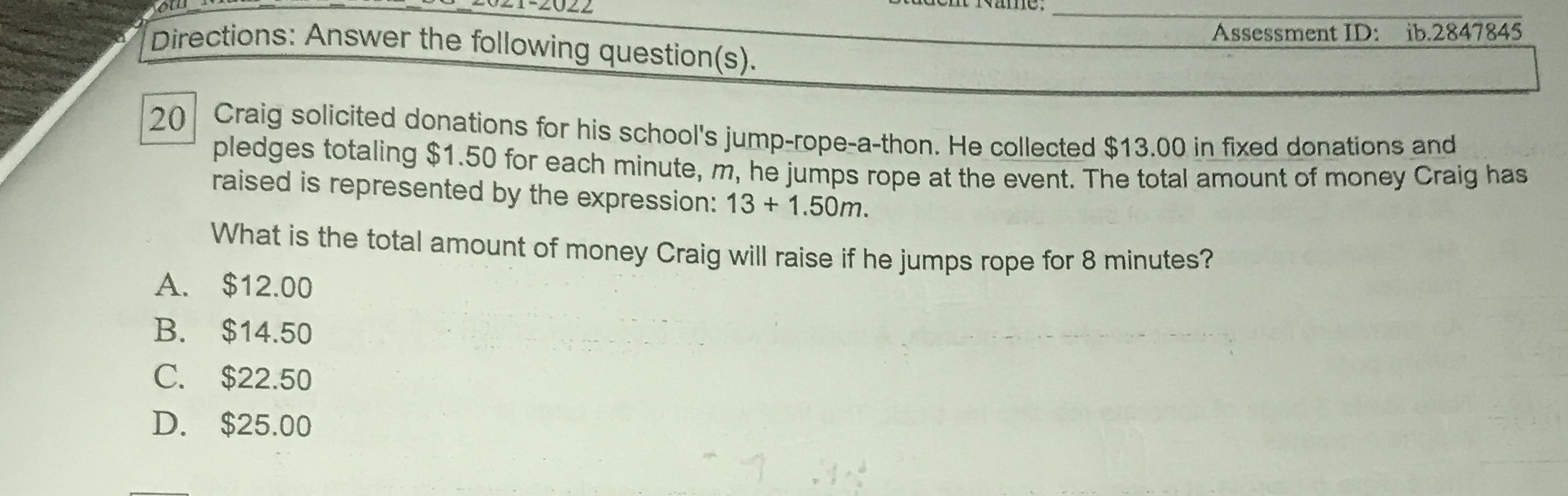### ¿Todavía tienes preguntas de matemáticas?

Pregunte a nuestros tutores expertos
Algebra
Pregunta$$20$$ Craig solicited donations for his school's jump-rope-a-thon. He collected $$\ 13.00$$ in fixed donations and pledges totaling $$\ 1.50$$ for each minute, $$m ,$$ he jumps rope at the event. The total amount of money Craig has raised is represented by the expression: $$13 + 1.50 m .$$

What is the total amount of money Craig will raise if he jumps rope for $$8$$ minutes?

A. $$\ 12.00$$

B. $$\ 14.50$$

C. $$\ 22.50$$

D. $$\ 25.00$$

D. $$\ 25.00$$